# Google Code Jam Archive — World Finals 2022 problems

## Overview

Code Jam 2022 World Finals were exciting until the last second, with many changes at the top of the scoreboard. The problems proved challenging as no problem was solved by everyone, and nobody solved every problem. The first problem, Wonderland Chase, was a classical graph theory problem with a novel observation. Goose, Goose, Ducks? came after as a logic-based problem, something unusual in programming contests. Then, Slide Parade seemed to be about paths, but ended up being a matching problem. The two most valuable problems were Schrödinger and Pavlov, a very unusual dynamic programming problem that not many contestants could solve, and Triangles, a hard geometry problem with a simple statement and a complicated solution that no contestant managed to find in time.

We hope that Code Jam 2022 was fun for everyone and gave you a new opportunity to sharpen your problems solving skills. We look forward to seeing everyone back again in 2022 for our twentieth anniversary! Until then, you can keep practicing on our platform with Kick Start.

Cast

Wonderland Chase: Written by Onufry Wojtaszczyk. Prepared by Shubham Avasthi.

Schrödinger and Pavlov: Written by Takuto Shigemura and Yui Hosaka. Prepared by Ikumi Hide.

Goose, Goose, Ducks?: Written by Onufry Wojtaszczyk. Prepared by John Dethridge.

Slide Parade: Written by Han-sheng Liu. Prepared by Ian Tullis and Yang Xiao.

Triangles: Written by Pablo Heiber. Prepared by Pablo Heiber and Timothy Buzzelli.

Solutions and other problem preparation and review by Artem Iglikov, Bakuri Tsutskhashvili, Bohdan Pryshchenko, Darcy Best, Harsh Lal, Hsin-Yi Wang, Ian Tullis, Jayasurya, John Dethridge, Jose Toro, Lazar Todorovic, Luis Santiago Re, Md Mahbubul Hasan, Mohamed Yosri Ahmed, Nafis Sadique, Nikos Papaspyrou, Nour Yosri, Pablo Heiber, Pawel Zuczek, Petr Mitrichev, Pi-Hsun Shih, Rayan Dasoriya, Raymond Kang, Salma Mustafa, Sanchit Malhotra, Sasha Fedorova, Sergiu Pușcaș, Timothy Buzzelli, Ulises Mendez Martinez, Viktoriia Kovalova, Vinay Khilwani, and Yan Li.

Analysis authors:

• Wonderland Chase: Max Ward.
• Goose, Goose, Ducks?: Pablo Heiber.
• Schrödinger and Pavlov: Pablo Heiber.
• Triangles: Pablo Heiber.

## A. Wonderland Chase

### Problem

Alice is trapped in Wonderland's labyrinth, being chased by the Queen of Hearts and her herald! The labyrinth is a set of $$\mathbf{J}$$$junctions numbered $$1$$$ through $$\mathbf{J}$$$, connected by $$\mathbf{C}$$$ bidirectional corridors.

Alice and the Queen of Hearts take turns making moves, and each knows the location of the other at all times. A move (by either of them) consists of either staying at the current junction or moving to another one that is connected to it by a corridor.

The Queen's herald, however, announces the next move the Queen makes in advance. That means that before anyone makes a move, he announces the Queen's first move. Then, Alice moves first. Then, each time the Queen moves, she must respect the previous announcement, and then decide her next move so the herald can announce it. Alice hears the announcements, so she always knows the Queen's next move before making her own.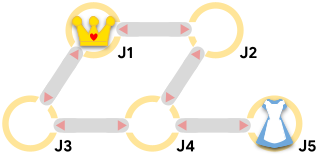### Output

For each test case, output one line containing Case #$$x$$$: $$y$$$, where $$x$$$is the test case number (starting from 1) and $$y$$$ is SAFE if Alice can avoid being caught for $$10^9$$$total moves. Otherwise, $$y$$$ is the total number of moves (including Alice's and the Queen's) that it takes for the Queen to catch Alice.

### Limits

Memory limit: 1 GB.
$$1 \le \mathbf{T} \le 100$$$. $$1 \le \mathbf{A} \le \mathbf{J}$$$.
$$1 \le \mathbf{Q} \le \mathbf{J}$$$. $$\mathbf{A} \ne \mathbf{Q}$$$.
$$1 \le \mathbf{U_i} \lt \mathbf{V_i} \le \mathbf{J}$$$, for all $$i$$$.
$$(\mathbf{U_i}, \mathbf{V_i}) \ne (\mathbf{U_j}, \mathbf{V_j})$$$, for all $$i \ne j$$$.

#### Test Set 1 (Visible Verdict)

Time limit: 10 seconds.
$$2 \le \mathbf{J} \le 30$$$. $$1 \le \mathbf{C} \le 60$$$.

#### Test Set 2 (Hidden Verdict)

Time limit: 60 seconds.
$$2 \le \mathbf{J} \le 10^5$$$. $$1 \le \mathbf{C} \le 2 \times 10^5$$$.

### Sample

Sample Input
4
5 5 5 1
1 2
1 3
2 4
3 4
4 5
5 5 5 2
1 2
1 3
2 4
3 4
4 5
3 1 2 3
1 3
2 1 1 2
1 2

Sample Output
Case #1: SAFE
Case #2: 4
Case #3: SAFE
Case #4: 2


Sample Case #1 is the one pictured in the problem statement. Alice's optimal first move is to move to junction $$4$$$. Sample Case #2 is the same as Sample Case #1 but the Queen starts at junction $$2$$$. The Queen can catch Alice by first announcing a move to junction $$4$$$. If Alice were to move to junction $$4$$$⁠, she would be caught in $$2$$$moves. Alice can evade capture for an extra $$2$$$ moves by staying put and waiting until the Queen then moves to junction $$5$$$where she is located.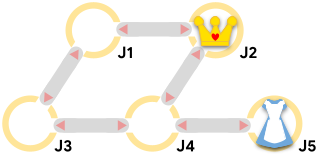In Sample Case #3, the Queen cannot reach Alice no matter what she does.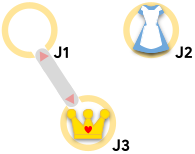In Sample Case #4, the Queen can begin by announcing that she will move to Alice's current junction. Alice has to move before then. If Alice moves to where the Queen already is, she gets caught immediately; if Alice remains in place, then she gets caught when the Queen moves. The second option is better, since it requires $$2$$$ total moves (Alice's and the Queen's) instead of $$1$$$.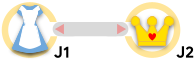## B. Goose, Goose, Ducks? ### Problem The first international Geese conference just wrapped up, and even though it should have been a happy occasion, it was bittersweet. The organizers found a paper with detailed plans of a duck infiltration. Now, they are trying to identify the infiltrating group from among the attendees. The document that they found contained a list of $$\mathbf{M}$$$ triples of integers $$(\mathbf{X_i}, \mathbf{Y_i}, \mathbf{C_i})$$$meaning the ducks would meet exactly $$\mathbf{C_i}$$$ seconds after the start of the conference at point $$(\mathbf{X_i}, \mathbf{Y_i})$$$, which is $$\mathbf{X_i}$$$ meters east and $$\mathbf{Y_i}$$$meters north of the center of the conference floor. Each goose may or may not have been at those specific points at those specific times, but every duck certainly was. Both ducks and geese walk at a maximum speed of one meter per second, which means an attendee that is at point $$(x, y)$$$ at time $$t$$$can reach any point of the form $$(x + \Delta_{x}, y + \Delta_{y})$$$ by time $$t + \Delta_{t}$$$as long as $${\Delta_{x}}^2 + {\Delta_{y}}^2 \le {\Delta_{t}}^2$$$. Each attendee's position at time $$0$$$can be any point, independently of the other attendees.After the discovery, the group held a questioning session to try to identify the ducks. During that session, attendees issued a series of statements, one at a time. The $$j$$$-th of those, in the order they were issued, was made by attendee $$\mathbf{A_j}$$$, claiming that both they and attendee $$\mathbf{B_j}$$$ were at point $$(\mathbf{U_j}, \mathbf{V_j})$$$exactly $$\mathbf{D_j}$$$ seconds after the start of the conference. Points in statements may or may not be points where duck meetings happened.

Statements from geese are always true, but ducks may lie. Moreover, ducks know which attendees are ducks and which are geese. To avoid getting caught easily, ducks only make statements that are consistent with all statements previously made by geese. Note that statements made by geese are consistent with all ducks being at all duck meetings.

It may not be possible to determine all the ducks with the information provided. However, knowing the minimum number of ducks will at least provide a lower bound on the level of duck activity. Note that there was at least one duck. Find this minimum number of ducks.

Formally, a hypothesis $$H$$$is a partition of all attendees into a set of ducks (named $$H$$$-ducks) and geese (named $$H$$$-geese). $$H$$$ is consistent with a set of statements $$S$$$if there exists a path for each attendee moving at most one meter per second such that: • all $$H$$$-ducks were at all duck meetings and
• for each statement in $$S$$$claiming that $$A$$$ saw $$B$$$at point $$P$$$ at time $$T$$$, both $$A$$$ and $$B$$$⁠'s paths went through point $$P$$$ at time $$T$$$. A hypothesis $$H$$$ is feasible under a set of statements $$S$$$if: • $$H$$$-ducks is not empty (i.e., there was at least one duck),
• the subset of all statements from $$S$$$made by members of $$H$$$-geese is consistent with $$H$$$(i.e., statements from geese are always true), and • for each statement $$s \in S$$$ made by a member of $$H$$$-ducks, if $$P \subseteq S$$$ is the subset of statements made by members of $$H$$$-geese issued before $$s$$$, there exists a hypothesis $$H'$$$(which may or may not be equal to $$H$$$) such that $$\{ s \} \cup P$$$is consistent with $$H'$$$ (i.e., ducks do not contradict previous statements made by geese).

Notice that the hypotheses $$H$$$such that $$H$$$-ducks contains all attendees is always feasible.

Find the minimum size of $$H$$$-ducks over all feasible hypotheses $$H$$$.

The first line of the input gives the number of test cases, $$\mathbf{T}$$$. $$\mathbf{T}$$$ test cases follow. Each test case starts with a line containing three integers, $$\mathbf{N}$$$, $$\mathbf{M}$$$, and $$\mathbf{S}$$$, representing the numbers of attendees, duck meetings, and statements, respectively. The next $$\mathbf{M}$$$ lines each describe a different duck meeting with three integers $$\mathbf{X_i}$$$, $$\mathbf{Y_i}$$$, and $$\mathbf{C_i}$$$, representing that there was a meeting at point $$(\mathbf{X_i}, \mathbf{Y_i})$$$, held exactly $$\mathbf{C_i}$$$seconds after the start of the conference. Then, the last $$\mathbf{S}$$$ lines of a test case each describe a statement. The $$j$$$-th of these lines describes the $$j$$$-th issued statement with five integers $$\mathbf{A_j}$$$, $$\mathbf{B_j}$$$, $$\mathbf{U_j}$$$, $$\mathbf{V_j}$$$, and $$\mathbf{D_j}$$$, representing that attendee $$\mathbf{A_j}$$$ stated that they and attendee $$\mathbf{B_j}$$$were both at point $$(\mathbf{U_j}, \mathbf{V_j})$$$ exactly $$\mathbf{D_j}$$$seconds after the start of the conference. ### Output For each test case, output one line containing Case #$$x$$$: $$y$$$, where $$x$$$ is the test case number (starting from 1) and $$y$$$is the minimum number of ducks that might have infiltrated the conference. ### Limits Memory limit: 1 GB. $$1 \le \mathbf{T} \le 50$$$.
$$-10^9 \le \mathbf{X_i} \le 10^9$$$, for all $$i$$$.
$$-10^9 \le \mathbf{Y_i} \le 10^9$$$, for all $$i$$$.
$$1 \le \mathbf{C_i} \le 10^9$$$, for all $$i$$$.
$$\mathbf{C_i} \lt \mathbf{C_{i+1}}$$$, for all $$i$$$.
$$(\mathbf{X_i} - \mathbf{X_{i+1}})^2 + (\mathbf{Y_i} - \mathbf{Y_{i+1}})^2 \le (\mathbf{C_i} - \mathbf{C_{i+1}})^2$$$, for all $$i$$$.
$$1 \le \mathbf{A_j} \le \mathbf{N}$$$, for all $$j$$$.
$$1 \le \mathbf{B_j} \le \mathbf{N}$$$, for all $$j$$$.
$$\mathbf{A_j} \ne \mathbf{B_j}$$$, for all $$j$$$.
$$-10^9 \le \mathbf{U_j} \le 10^9$$$, for all $$j$$$.
$$-10^9 \le \mathbf{V_j} \le 10^9$$$, for all $$j$$$.
$$1 \le \mathbf{D_j} \le 10^9$$$, for all $$j$$$.
$$(\mathbf{A_j}, \mathbf{B_j}, \mathbf{U_j}, \mathbf{V_j}, \mathbf{D_j}) \ne (\mathbf{A_k}, \mathbf{B_k}, \mathbf{U_k}, \mathbf{V_k}, \mathbf{D_k})$$$, for all $$j \ne k$$$.

#### Test Set 1 (Visible Verdict)

Time limit: 20 seconds.
$$2 \le \mathbf{N} \le 50$$$. $$1 \le \mathbf{M} \le 50$$$.
$$1 \le \mathbf{S} \le 50$$$. #### Test Set 2 (Hidden Verdict) Time limit: 60 seconds. $$2 \le \mathbf{N} \le 10^5$$$.
$$1 \le \mathbf{M} \le 10^5$$$. $$1 \le \mathbf{S} \le 10^5$$$.

### Sample

Sample Input
2
2 1 2
1 2 3
1 2 1 1 1
2 1 2 2 2
4 2 4
4 3 10
-4 -3 20
1 3 4 3 11
2 4 0 0 16
3 1 6 3 9
4 2 0 0 16

Sample Output
Case #1: 1
Case #2: 2


In Sample Case #1, attendee 1 being the only duck is a feasible hypothesis.

In Sample Case #2, attendees 2 and 4 being the only ducks is a feasible hypothesis. Note that there is at least one duck, so all attendees being geese is not feasible.

### Problem

Gooli is a huge company that owns $$\mathbf{B}$$$buildings in a hilly area, numbered $$1$$$ through $$\mathbf{B}$$$. Six years ago, Gooli built slides that allowed employees to go from one building to another. Each slide allows anyone to go from the slide's origin building to the slide's destination building, but not the other way around. Gooli's CEO is very proud of their slides and wants to organize a parade through the slides. She has tasked Melek, Gooli's Head of Transportation and a problem-solving enthusiast, with designing the parade's route.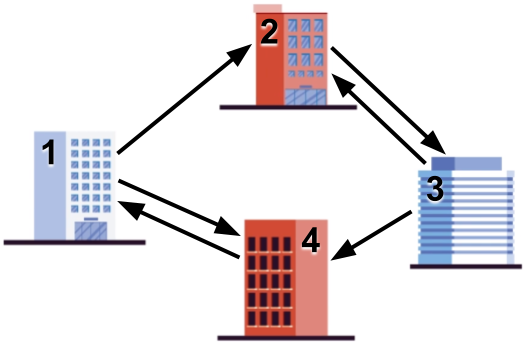She has some requirements for the parade route in mind: • It must start and end at building $$1$$$, where her office is located.
• It must visit each building the same number of times. Being in building $$1$$$at the start of the route does not count as a visit. • It must use each slide at least once. • It must have at most $$10^6$$$ steps.

Given the layout of buildings and slides, help Melek find a route that satisfies all of the CEO's requirements, if one exists.

The first line of the input gives the number of test cases, $$\mathbf{T}$$$. $$\mathbf{T}$$$ test cases follow. Each test case starts with a line containing two integers $$\mathbf{B}$$$and $$\mathbf{S}$$$: the number of buildings and slides, respectively. Then, $$\mathbf{S}$$$lines follow. The $$i$$$⁠-⁠th of these lines contains two integers $$\mathbf{U_i}$$$and $$\mathbf{V_i}$$$, indicating that the $$i$$$⁠-⁠th slide goes from building $$\mathbf{U_i}$$$ to building $$\mathbf{V_i}$$$. ### Output For each test case, output one line containing Case #$$x$$$: $$y$$$, where $$x$$$ is the test case number (starting from 1). If there is no route that fulfills all the requirements, $$y$$$must be IMPOSSIBLE. If there is, $$y$$$ must be an integer between $$\mathbf{S}+1$$$and $$10^6+1$$$, inclusive, representing the length of one such route you want to exhibit. In that case, output another line containing $$y$$$integers $$z_1\ z_2\ \dots\ z_y$$$, where $$z_j$$$is the $$j$$$⁠-⁠th building in your proposed route. Notice that $$z_1 = z_y = 1$$$and that each building must appear the same number of times among the $$z_j$$$, except for building $$1$$$, which appears exactly one extra time. ### Limits Memory limit: 1 GB. $$1 \le \mathbf{T} \le 100$$$.
$$1 \le \mathbf{U_i} \le \mathbf{B}$$$, for all $$i$$$.
$$1 \le \mathbf{V_i} \le \mathbf{B}$$$, for all $$i$$$.
$$\mathbf{U_i} \ne \mathbf{V_i}$$$, for all $$i$$$.
$$(\mathbf{U_i}, \mathbf{V_i}) \neq (\mathbf{U_j}, \mathbf{V_j})$$$, for all $$i \neq j$$$.

#### Test Set 1 (Visible Verdict)

Time limit: 10 seconds.
$$2 \le \mathbf{B} \le 10$$$. $$2 \le \mathbf{S} \le 10$$$.

#### Test Set 2 (Hidden Verdict)

Time limit: 20 seconds.
$$2 \le \mathbf{B} \le 200$$$. $$2 \le \mathbf{S} \le 5000$$$.

### Sample

Sample Input
5
2 2
2 1
1 2
3 4
2 3
1 2
3 2
1 3
3 6
1 2
1 3
2 1
2 3
3 1
3 2
3 4
1 2
2 1
1 3
3 1
4 6
1 2
1 4
2 3
3 2
3 4
4 1

Sample Output
Case #1: 7
1 2 1 2 1 2 1
Case #2: IMPOSSIBLE
Case #3: 7
1 2 3 1 3 2 1
Case #4: IMPOSSIBLE
Case #5: 9
1 4 1 2 3 2 3 4 1


In Sample Case #1, another acceptable parade route is one that goes from building $$1$$$to building $$2$$$ and then back for a total of $$2$$$steps.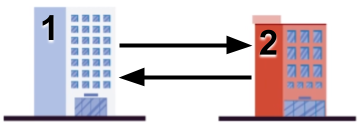In Sample Case #2, there are no slides leading to building $$1$$$, so no valid parade can exist.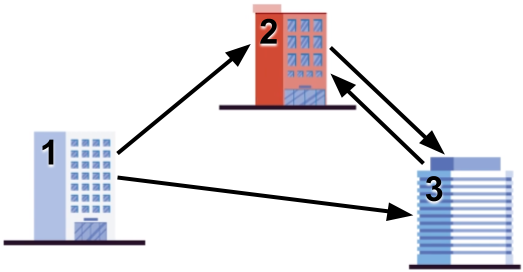In Sample Case #3, the parade route the sample output exhibits goes through each building twice.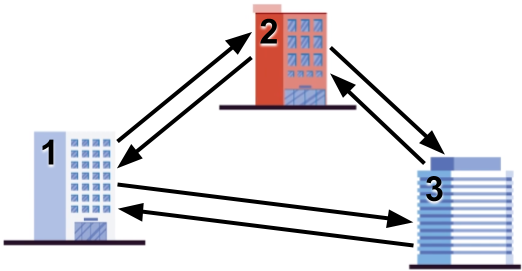Sample Case #4 is pictured below.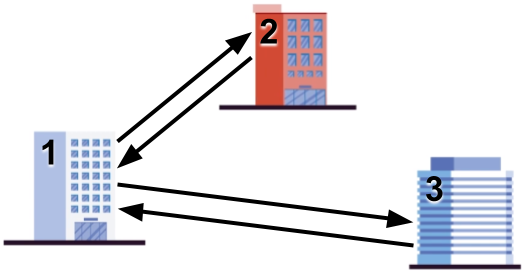Sample Case #5 is the one illustrated in the problem statement. In the parade route in the sample output, the slides from $$2$$$to $$3$$$ and from $$4$$$to $$1$$$ are used twice, but the rest of the slides are used only once each.

## D. Schrödinger and Pavlov

### Problem

The story, all names, characters, and incidents portrayed in this problem statement are fictitious. No identification with actual persons is intended or should be inferred.

It is 1935 and a meeting between two Nobel prize winners is producing astonishing results. Schrödinger, a famous physicist, invited Pavlov, a famous physiologist, to see his experiments with cats in boxes. Pavlov brought his dog with him to keep up with his own research, and the combination proved interesting, to say the least.

Schrödinger had a row of $$\mathbf{N}$$$boxes. Some boxes definitely contain a cat, some boxes definitely do not contain a cat, and some boxes may or may not contain a cat. Each box is only big enough to hold a single cat. Each box is also equipped with a special quantum tunnel, that allows the cat in the box to move to some other specific box if the destination was empty. The tunnels work in a single direction. Cats are usually mellow and quiet and do not use the tunnels unless they become startled. When a third unannounced guest rings the bell, Pavlov's dog gets excited immediately and starts running and barking. The dog starts at box $$1$$$ and runs towards box $$\mathbf{N}$$$. As the dog runs, it passes right next to each box, one at a time. When it passes next to a box that contains a cat, the cat in that box becomes startled. The startled cat checks the available tunnel and, if the destination box is empty, uses it to escape. If the destination box is occupied, the cat stays in its current box. The same cat can be startled more than once if they move to a box the dog will get to afterwards, and will proceed in the same way every time it is startled (using only the newly available tunnel each subsequent time).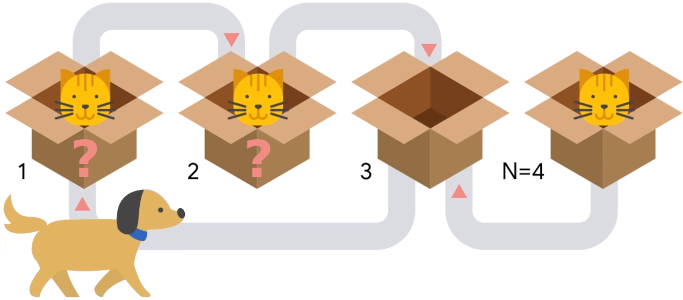After Pavlov's dog finally stops right next to the last box, Pavlov asks Schrödinger whether there is a cat in that last box. Schrödinger, true to his fame, replies that he does not know. Pavlov notices that the answer may depend on whether or not there were cats in the unknown boxes. Moreover, he also notices that because there are $$k$$$ unknown boxes, there are $$2^k$$$possible initial configurations, one for each combination of statuses of the unknown boxes. Pavlov tells Schrödinger that they should try to calculate how many of the $$2^k$$$ initial configurations would result in having a cat in the last box. You are asked to recreate that calculation. Since the output can be a really big number, we only ask you to output the remainder of dividing the result by the prime $$10^9+7$$$($$1000000007$$$).

Neither cats, nor dogs, nor Nobel prize winners were harmed in the making of this problem statement.

The first line of the input gives the number of test cases, $$\mathbf{T}$$$. $$\mathbf{T}$$$ test cases follow, each described by exactly three lines. The first line of a test case contains a single integer $$\mathbf{N}$$$, the number of boxes in Schrödinger's experiment. Boxes are numbered between $$1$$$ and $$\mathbf{N}$$$, in the order Pavlov's dog passes them by. The second line of a test case contains a single string $$\mathbf{S}$$$ of $$\mathbf{N}$$$characters. The $$i$$$-th character of $$\mathbf{S}$$$(counting from left to right) represents the contents of box $$i$$$: it is an uppercase 'C' if the box contains a cat, a period '.' if the box does not contain a cat and a question mark '?' if it is unknown whether the box contains a cat or not. The third line of a test case contains $$\mathbf{N}$$$integers $$\mathbf{B_1}, \mathbf{B_2}, \dots, \mathbf{B_N}$$$, representing that there is a tunnel going out of box $$i$$$and into box $$\mathbf{B_i}$$$, for all $$i$$$. ### Output For each test case, output one line containing Case #$$x$$$: $$y$$$, where $$x$$$ is the test case number (starting from 1) and $$y$$$is the number of initial configurations that would result in a cat being in the last box and unable to escape despite hearing the barking, modulo the prime $$10^9+7$$$ ($$1000000007$$$). ### Limits Time limit: 10 seconds. Memory limit: 1 GB. $$1 \le \mathbf{T} \le 1234$$$.
the length of $$\mathbf{S} = \mathbf{N}$$$. Each character of $$\mathbf{S}$$$ is either an upper case 'C', a period '.' or a question mark '?'.
$$1 \le \mathbf{B_i} \le \mathbf{N}$$$, for all $$i$$$.
$$\mathbf{B_i} \neq i$$$, for all $$i$$$.

### Sample

Sample Input
4
4
??.C
2 3 1 3
4
????
2 3 1 3
6
?.????
6 6 6 6 6 5
34
????????????????????????????????CC
2 3 4 5 6 7 8 9 10 11 12 13 14 15 16 17 18 19 20 21 22 23 24 25 26 27 28 29 30 31 32 33 34 33

Sample Output
Case #1: 1
Case #2: 2
Case #3: 15
Case #4: 294967268


Sample Case #1 is illustrated in the problem statement. There are $$4$$$possible configurations: • ...C: the dog runs through the first $$3$$$ boxes without changing anything because there is no cat there. Then, when it gets to the last box, the cat hears it and escapes to box 3. Therefore, there is no cat in the last box in this case.
• C..C: when the dog barks near box $$1$$$, that startles the cat that goes through the tunnel to get to box $$2$$$, which was empty. Then, the same cat gets startled again when the dog barks near box $$2$$$and gets to box $$3$$$. And when the dog barks next to box $$3$$$, the cat hears it and returns to box $$1$$$. Therefore, when the dog gets to box $$4$$$and the other cats hears it, box $$3$$$ is empty so the cat escapes and the last box ends up empty.
• .C.C: This case is very similar to the previous one. After the dog goes through the first box and nothing happens, the state is the same as before, so the ultimate result is the same: last box empty.
• CC.C: In this case, the cat in the first box cannot escape when it hears the dog, so it remains in box $$1$$$. Then, when the cat in box $$2$$$ gets startled it escapes to box $$3$$$leaving a state of C.CC. When the dog gets to the box $$3$$$, the cat currently there cannot escape to box $$1$$$so the state remains the same. Finally, when the dog gets to the last box, the cat that is there cannot escape because box $$3$$$ is occupied this time. So, in this case, the last box ends up with a cat after the dog ends its journey.
Out of the $$4$$$possibilities, only $$1$$$ (the last one) ends up with a cat in the last box, so the answer is $$1$$$. In Sample Case #2, the tunnels are set up the same as in Sample Case #1. Since no tunnel ends at the last box, the configurations that start with no cat at the last box will also not end with a cat there, so we do not need to count them. Then, we have $$8$$$ additional configurations. The $$4$$$we considered for Sample Case #1, out of which only $$1$$$ ends up with a cat at the last box. The remaining $$4$$$configurations are: ..CC, C.CC, .CCC, CCCC. From these additional $$4$$$ configurations, only in the last one listed a cat ends up in the last box, for a total of $$2$$$overall. In Sample Case #3, notice that for a cat to remain in the last box after the dog barks near it, both that box and box $$5$$$ must be occupied then (otherwise, either there is no cat in the last box, or it will escape to box $$5$$$). Since there is no tunnel going into box $$5$$$, a cat must start there. As long as there is another cat in any other box, box $$6$$$will get (or remain) occupied before the cat in box $$5$$$ gets an opportunity to escape, so all of those will end up with a cat in the last box. As we argued before, a single cat is not enough. Thus, we need to count the number of configurations with a cat in box $$5$$$and at least one other cat. There are $$2^4$$$ configurations with a cat in box $$5$$$, and out of those, only $$1$$$ has no other cat, so the answer is $$2^4-1=15$$$. In Sample Case #4, in all of the $$2^k$$$ ways in which the $$k$$$unknown boxes may exist a cat would be left in the last box. ## E. Triangles ### Problem You are given a set $$P$$$ of $$\mathbf{N}$$$distinct points in the two-dimensional plane. You want to find a maximum set of triangles such that: • Each vertex of a triangle in your set is a point from $$P$$$ and each point in $$P$$$is a vertex of at most one triangle in your set. • Each triangle in your set has positive area (i.e., its $$3$$$ vertices are not collinear).
• For any two sides of triangles in your set, their intersection is either empty or an endpoint of one of them.
• For any two triangles in your set, the intersection of the areas strictly inside those triangles is either empty or equal to one of them.

For example, the set of triangles depicted below meets the definition above.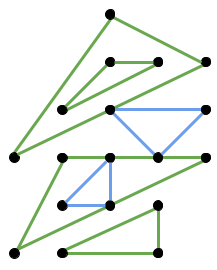On the other hand, each pair of a yellow and a red triangle in the picture below does not meet the definition.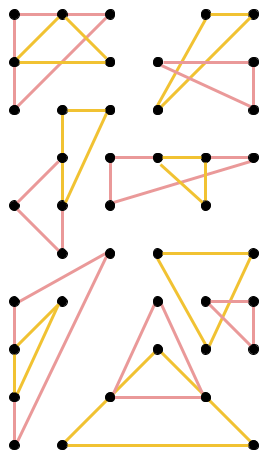### Input

The first line of the input gives the number of test cases, $$\mathbf{T}$$$. $$\mathbf{T}$$$ test cases follow. Each test case starts with a line containing a single integer $$\mathbf{N}$$$. Then, $$\mathbf{N}$$$ lines follow. The $$i$$$-th of these lines contains two integers $$\mathbf{X_i}$$$ and $$\mathbf{Y_i}$$$representing the coordinates of the $$i$$$-th point.

### Output

For each test case, output one line containing Case #$$x$$$: $$y$$$, where $$x$$$is the test case number (starting from 1) and $$y$$$ is the maximum size of a set of triangles with the desired properties. Then, output $$y$$$more lines. The $$j$$$-th of those lines must contain $$p_j\ q_j\ r_j$$$representing that the $$j$$$⁠-⁠th triangle in your proposed set has the $$p_j$$$-th, $$q_j$$$-th, and $$r_j$$$-th points in the input as vertices. Points in the input are numbered starting from $$1$$$.

### Limits

Time limit: 15 seconds.
Memory limit: 1 GB.

$$3 \le \mathbf{N} \le 3000$$$. ### Sample Sample Input 3 9 8 2 10 2 2 0 0 5 2 3 10 4 10 0 8 3 2 4 7 0 0 0 3 3 0 0 1 1 0 1 1 2 2 3 0 0 0 1 0 2  Sample Output Case #1: 3 3 4 5 1 7 9 6 2 8 Case #2: 2 2 3 1 6 5 4 Case #3: 0  Sample Case #1 is illustrated below. Notice that there are other valid ways to construct a maximum number of triangles.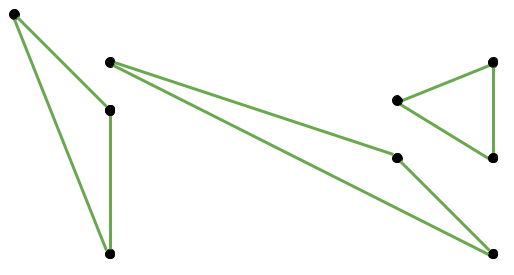Sample Case #2 is illustrated below. As before, there are other valid ways to construct $$2$$$ triangles.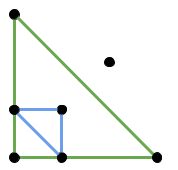### Test Set 2

A faster solution is needed for Test Set 2. We will need some more observations. Call a node good when, if Alice reaches it before being caught, Alice will always be safe. That is, if Alice reaches a good node, then regardless of where the Queen is, Alice can always move in a way that is safe. Let's consider an algorithm for finding all good nodes.

Assuming a connected graph, leaves (nodes with degree 1) are never good because Alice can become cornered in them. We can start by deleting them. In fact, if we iteratively remove leaves until there are none left, then all the remaining nodes must be good, since Alice can never be cornered. This algorithm can be implemented in linear time using a queue of leaves and keeping track of the degree of each node throughout deletions.

Define $$\mathbf{DA}_u$$$as the shortest path from Alice's starting node to a node $$u$$$. Likewise, define $$\mathbf{DQ}_u$$$as the shortest path from the Queen. For a node $$j$$$, if $$\mathbf{DA}_j < \mathbf{DQ}_j$$$, then Alice can safely reach that node before being caught. We can prove this using a contradiction: if the Queen was able to intercept Alice anywhere on her path, then the Queen must have reached some node on Alice's shortest path before Alice, which would imply that the Queen can reach $$u$$$ first. Note that $$\mathbf{DA}$$$and $$\mathbf{DQ}$$$ can be computed by running one Breadth-First Search (BFS) each and storing the resulting table of distances.

We can use our observations to solve the problem by considering a few cases. Alice will be safe if:

• The graph is disconnected, meaning Alice and the queen start in two disconnected components. This can be checked by looking at either $$\mathbf{DA}$$$or $$\mathbf{DQ}$$$.
•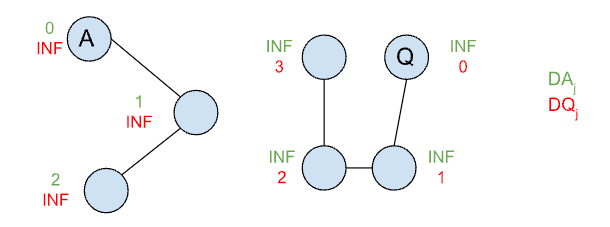• Alice can enter a good node $$j$$$such that $$\mathbf{DA}_j < \mathbf{DQ}_j$$$.
•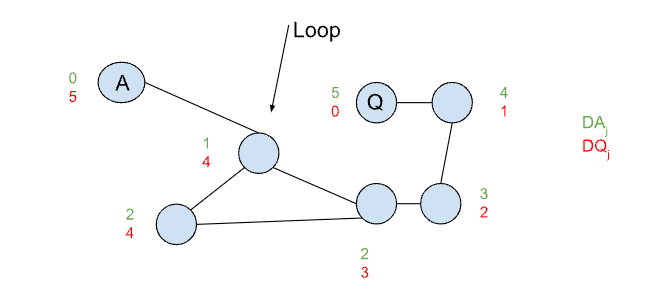Otherwise, Alice will get caught. Since Alice's strategy is to maximize the number of moves until she is caught, she will pick the junction that has the maximum distance from the Queen with the condition that she can get to it first ($$\mathbf{DA}_j < \mathbf{DQ}_j$$$).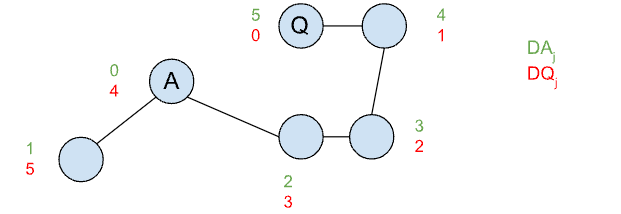Since the solution requires only three linear passes over the graph (one to find good nodes, and two BFS runs), the total complexity is $$O(\mathbf{J} + \mathbf{C})$$$.

Test Data
info We recommend that you practice debugging solutions without looking at the test data.

## Analysis — B. Goose, Goose, Ducks?

### Test Set 1

Consistency in this problem has a property which is not true for most typical logic systems, in that a set of statements is consistent if and only if each subset of size $$2$$$of it is consistent. The left to right implication is trivial, but the converse is not, so let us prove it. Assume you have a set of statements $$S$$$ such that any two of them are consistent. Then, for each bird $$b$$$, consider all statements that state that $$b$$$ is at a specific point in time and space. If we sort all those points by time and linearly interpolate between consecutive points, we obtain a path for $$b$$$that is consistent with all statements in $$S$$$. Notice that, because any pair of statements is consistent — in particular, pairs of consecutive statements — the linear interpolation part does not exceed the maximum speed. In this way, we can obtain a path for each mentioned bird, all of which are consistent with all statements. Then, by definition, $$S$$$is consistent. Meetings also state "this bird was at this time-space point" for birds that are ducks. Thus, an analogous reasoning shows that a set of statements and known ducks is consistent with the meetings if and only if they are pairwise consistent. Since the meetings are pairwise consistent according to the limits, this leaves only pairs of two statements and pairs of a statement a meeting to check. At this point, with the limits in Test Set 1 being so small, we can simply try everything. We know there is at least one duck, so start by trying every bird as "the first duck". Within each option, go through statements and check them against meetings (if they involve a known duck) and against previous statements. If a statement contradicts a meeting, the issuer of that statement must be a duck. If a statement contradicts a previous statement, then the issuer of such previous statement must be a duck (since ducks cannot contradict geese). Each time we find a new duck, we start checking everything again. When we go through all statements without finding any contradictions with our current set of ducks, we are done and we have a candidate set of ducks. Notice that all birds being ducks is always a valid answer. Finally, we keep the smallest set of ducks and output its size. This solution requires $$\mathbf{N}$$$ iterations of the outer loop, to try every possible "first duck". Checking a pair of statements or a statement and a meeting for consistency takes constant time, as it is only checking whether birds that are mentioned in both can get from one point in space-time to another, which is a simple bit of math. Thus, checking every statement against every other statement and every meeting takes $$O(\mathbf{S} \times (\mathbf{S} + \mathbf{M}))$$$. On every iteration through statements except for one (the last one) we find at least one additional duck, so there are at most $$\mathbf{N} - 1$$$ iterations (remember we start with an identified duck). Therefore, the overall running time is $$O(\mathbf{N}^2 \times \mathbf{S} \times (\mathbf{S} + \mathbf{M}))$$$. With all those variables being bounded by $$50$$$, that should fit in time.

### Test Set 2

In Test Set 2, we need to speed up things significantly. We can start by making use of a more refined version of our consistency observations. As you can see in the proof, we do not need to require every pair of statements or a statement and a meeting to be consistent: only those that are "consecutive".

Formally, let us call two statements $$s_1$$$and $$s_2$$$ consecutive in $$S$$$if they refer to times $$t_1$$$ and $$t_2$$$, respectively, and to bird $$b$$$, and there is no other statement in $$S$$$that refers to a time $$t_3$$$ such that $$t_1 \lt t_3 \lt t_2$$$and to bird $$b$$$. Similarly, let us call a statement $$s$$$in $$S$$$ that refers to time $$t_1$$$and a meeting $$m$$$ at time $$t_2$$$consecutive if there is no other meeting at time $$t_3$$$ such that $$t_1 \lt t_3 \lt t_2$$$. Notice that consecutive is not a total order, because of statements referring to the same time. However, making it a total order by breaking ties arbitrarily maintains the validity of the theorem. Then, we can say that a set $$S$$$ of statements and/or meetings with known ducks is consistent if and only if any consecutive pair of them is consistent. The proof is the same as the one given above.

With the observation above, if we can maintain a sorted list of meetings and goose-made statements about each bird, we can check the consistency for each statement $$s$$$in logarithmic time by only checking its consecutive neighbors (at most $$2$$$ meetings and $$2$$$statements per bird in $$s$$$). This would already reduce the $$O(\mathbf{S} \times (\mathbf{S} + \mathbf{M}))$$$inner-most check in the Test Set 1 solution to logarithmic time, a significant improvement. To maintain that, we can keep a tree structure that allows insertion and lookup in logarithmic time for each bird (like set in C++ or TreeSet in Java). In this way, every time we process a statement, we simply add the new information to the appropriate birds. We can further improve by not resetting every time we find a duck. If our structure also allows removal in logarithmic time (like the examples above), we can do the following: When we discover a duck, delete all information coming from their statements. For that, we keep a list for each bird of all information they contributed. Since each piece of information is removed at most once, the overall number of removals is at most the overall number of insertions and does not affect the time complexity. At this point we have reduced the complexity to $$O(\mathbf{N} \times (\mathbf{S} + \mathbf{M}) \times F)$$$ where $$F$$$is only logarithmic factors. The $$\mathbf{N}$$$ comes from the external "first duck" iteration. To improve upon that, we can first notice that, if we start from the empty set of ducks and still find some, those birds must always be ducks, and would be ducks when starting from any set. Therefore, in that case, that set of ducks is the answer. If there are no forced ducks, we need to do something different, but we know there is no inconsistency between statements.

At this point, we only need to check consistency between statements and meetings. A statement being inconsistent with a meeting is equivalent to a bird $$b_1$$$saying that bird $$b_2$$$ is not a duck. Therefore, if $$b_2$$$were a duck, so would $$b_1$$$. We can represent the situation with a directed graph in which the edges represent these implications. If there is a path in that graph from $$b_1$$$to $$b_2$$$, then there is an implication (possibly not coming directly from a single statement) that if $$b_1$$$is a duck, then so is $$b_2$$$. So, if a bird is a duck, then so is every other bird in its strongly connected component (SCC). That means we want to choose an SCC that is as small as possible. Moreover, we want to choose a final component, that is, one that is not pointed to by other components. Non-final components imply other components also being made of ducks, and ultimately bringing in at least one final component. In summary, in this final case the answer is the size of the smallest final SCC, which we can find in linear time.

Test Data
info We recommend that you practice debugging solutions without looking at the test data.

## Analysis — C. Slide Parade

In this problem, we are given a simple graph $$G$$$. We are required to find a sufficiently-small circuit that passes through each edge at least once, and passes through all vertices the same number of times. Let the input graph be $$G = (V, E)$$$.
$$V = \{1, 2, ..., B\}$$$, $$E = \{(X_1, Y_1), (X_2, Y_2), ..., (X_S, Y_S)\}$$$.

If the circuit exists, let $$k$$$be the number of times that each vertex is passed through. Collecting the edges in the circuit forms a multigraph $$G' = (V, E')$$$, where $$E'$$$is a multiset. $$G'$$$ has the following properties:

• The underlying set of $$E'$$$is $$E$$$. Which means
• Each edge in $$E$$$appears at least once in $$E'$$$.
• Each edge in $$E'$$$appears in $$E$$$.
• For each node $$v$$$, $$indegree(v) = outdegree(v) = k$$$.

On the other hand, if we could find a multigraph $$G'$$$satisfying the above properties for some $$k$$$, then we could compute an answer from $$G'$$$with an Eulerian circuit construction algorithm. An Eulerian circuit is a circuit that visits each edge in a graph exactly once. In the following solutions, we will focus on finding a $$G'$$$ via different approaches.

Let $$L$$$be the output limit $$10^6$$$. Note that $$L$$$is also a significant factor while analyzing the complexity. ### $$O(L\mathbf{BS})$$$ approach

In this approach, we will use a max-flow algorithm to find a set of valid multiplicities $$d_i$$$for each $$(X_i, Y_i)$$$ in $$E$$$. i.e. $$(X_i, Y_i)$$$ appears $$d_i$$$times in $$E'$$$.

Note that the total number of edges in the cycle would be $$k\mathbf{B}$$$, so the upper bound of $$k$$$ is $$\dfrac{L}{\mathbf{B}}$$$. Enumerating all possible $$d_i$$$ is not feasible, but we can try all possible values of $$k$$$, and see if a set of valid $$d_i$$$ can be generated under the fixed $$k$$$. Once we find a set of valid $$d_i$$$, then $$G'$$$is also determined. This can be reduced to a maximum flow problem. We will construct a flow network with source $$s$$$ and sink $$t$$$to help us find a valid set of $$d_i$$$.

The vertices of the flow network are:

• The source $$s$$$. • The sink $$t$$$.
• A node $$in_v$$$for each $$v$$$ in $$V$$$. • A node $$out_v$$$ for each $$v$$$in $$V$$$.
There are $$(2\mathbf{B}+2)$$$vertices in the network in total. The edges of the flow network are: • $$(s, out_v)$$$ for each $$v$$$in $$V$$$, with capacity $$k$$$. • $$(out_u, in_v)$$$ for each $$(u, v)$$$in $$E$$$. No capacity limit, but the lower bound of flow is 1.
• $$(in_v, t)$$$for each $$v$$$ in $$V$$$, with capacity $$k$$$.
There are $$(\mathbf{S}+2\mathbf{B})$$$edges in the network in total. The amount of flow through $$in_v$$$ ($$out_v$$$, resp.) indicates the indegree (outdegree, resp.) of vertex $$v$$$ in $$G'$$$. The amount of flow on $$(out_u, in_v)$$$ indicates the multiplicity of edge $$(u, v)$$$. Now we search for the maximum flow on the graph. If the total flow is $$k\mathbf{B}$$$ (the maximum possible), then the amount of flow through $$in_v$$$and $$out_v$$$ is $$k$$$for each $$v$$$, and we have found a valid set of multiplicities $$d_i$$$. We can then construct $$G' = (V, E')$$$ with those multiplicities, find an Eulerian circuit in $$G'$$$, and output that circuit. On the other hand, if we don't find such a flow for any possible $$k$$$, then we output IMPOSSIBLE.

Now let's analyze the time complexity of this solution. In this solution, we run a maximum flow algorithm for each $$k$$$. There are $$\dfrac{L}{\mathbf{B}}$$$ possibilities of $$k$$$, and the graph has at most $$O(\mathbf{B})$$$ vertices and $$O(\mathbf{S})$$$edges. Thus the total time complexity is $$O(L\mathbf{BS})$$$, assuming Dinic's $$O(\mathbf{B^2S})$$$algorithm is chosen for solving maximum-flow. This can be made faster by computing a maximum flow for each $$k$$$ by starting with the flow found for the previous value of $$k$$$. ### $$O(\mathbf{S^2})$$$ approach

The previous algorithm could be somewhat slow. It would be good if we could generate a graph $$G'$$$more directly. Consider this simple iterative algorithm that produces a $$G'$$$:

• Choose an edge (u, v) that is not yet in $$G'$$$. • Add edges on a path from building 1 to building u to $$G'$$$.
• Add the edge (u, v) to $$G'$$$. • Add edges on a path from building v to building 1 to $$G'$$$.
• Repeat until each edge occurs at least once in $$G'$$$. The $$G'$$$ produced by this algorithm is able to produce a circuit, since we can simply follow the edges in the same order they were added. But the buildings would not necessarily be visited an equal number of times. So, it would be good if we could do the following instead:

• Choose an edge $$(u,v)$$$that is not yet in $$G'$$$.
• Find a set $$A$$$of edges, such that the indegree and outdegree of each node is equal in $$A$$$, and $$A$$$includes $$(u, v)$$$.
• Add $$A$$$to $$G'$$$.
• Repeat until each edge occurs at least once in $$G'$$$. If this succeeds, it would yield a $$G'$$$ with all the required properties we listed earlier. Also, if every set $$A$$$found in the algorithm was as small as possible, that is, if the indegree and outdegree of each node in each $$A$$$ was $$1$$$, then the total number of edges in $$G'$$$ would be at most $$SB$$$, which is at most $$10^6$$$, which conveniently is the limit in the problem!

Now, the question arises — if the problem is possible, can we always find such a set $$A$$$for any edge $$(u,v)$$$? We can show that we can.

The problem of finding a set $$A$$$of edges can be reduced to finding a perfect bipartite matching, on a graph similar to the flow graph in the previous solution. The bipartite graph consists of vertices $$(s_1, s_2, \dots s_\mathbf{B})$$$ and $$(t_1, t_2, \dots t_\mathbf{B})$$$. There is an edge $$(s_u, t_v)$$$ if and only if $$(u, v)$$$is in $$G$$$. If we use an edge in the perfect matching, then we add the corresponding edge in $$G$$$to $$A$$$.

We need to show that a perfect matching exists in this graph if the problem instance is possible. To do that, we apply Hall's marriage theorem, which is a common technique for proving whether a graph has a perfect matching.

What we need to prove to use the theorem is the following: if there is a solution to the problem, then for any subset $$S$$$of the nodes$$(s_1, s_2, \dots s_\mathbf{B})$$$, let $$T$$$be the set of all nodes adjacent to a node in $$S$$$. Then $$|S| \leq |T|$$$. (We must also show that a similar result holds if we start with a subset of the nodes $$(t_1, t_2, \dots t_\mathbf{B})$$$, but the proof for that is the same.)

Assume there is a solution to the problem, which has a corresponding multigraph $$G'$$$, in which the indegree and outdegree of each node is $$k$$$.

For each $$i$$$, let $$g(s_i)$$$ be the number of edges in $$G'$$$whose tail is node $$i$$$.

For each $$i$$$, let $$g(t_i)$$$ be the number of edges in $$G'$$$whose head is node $$i$$$.

Now for any pair of $$S$$$and $$T$$$ above, $$\begin{equation} \sum_{s \in S}{g(s)} \leq \sum_{t \in T}{g(t)} \end{equation}$$$since the edges in $$G'$$$ whose head is in $$T$$$must include all the edges in $$G'$$$ whose tail is in $$S$$$, plus possibly some more. But because $$G'$$$ corresponds to a solution, the values of $$g$$$must all be equal to $$k$$$. So we can conclude that $$|S| \leq |T|$$$as required. But this is not exactly the bipartite matching problem we need to solve - we need to include some fixed edge $$(s_u, t_v)$$$ in the matching each time. So remove all other edges adjacent to $$s_u$$$or $$t_v$$$ from the bipartite graph, and now define $$g$$$as follows: For each $$i$$$, let $$g(s_i)$$$be the number of edges in $$G'$$$ whose tail is node $$i$$$, excluding those edges deleted from the bipartite graph. For each $$i$$$, let $$g(t_i)$$$be the number of edges in $$G'$$$ whose head is node $$i$$$, excluding those edges deleted from the bipartite graph. Consider a set $$S$$$ which does not contain $$s_u$$$. $$\begin{equation} k|S|-k \lt \sum_{s \in S}{g(s)}\end{equation}$$$, since less than $$k$$$edges were removed from the graph. Also, $$\begin{equation} \sum_{t \in T}{g(t)} \leq k|T| \end{equation}$$$ since $$k$$$is still the maximum value of any $$g(t)$$$. We still have $$\begin{equation} \sum_{s \in S}{g(s)} \leq \sum_{t \in T}{g(t)} \end{equation}$$$as before, so $$\begin{equation} k|S|-k \lt \sum_{s \in S}{g(s)} \leq \sum_{t \in T}{g(t)} \leq k|T| \end{equation}$$$

$$\begin{equation}\therefore k|S|-k \lt k|T| \end{equation}$$$$$\begin{equation}\therefore |S|-1 \lt |T| \end{equation}$$$

$$\begin{equation}\therefore |S| \leq |T| \end{equation}$$$as required. The same result holds for sets $$S$$$ which do contain $$s_u$$$, since we simply match $$s_u$$$ with $$t_v$$$and are left with a set $$S$$$ without $$s_u$$$and we can proceed as above. If we fail to find a perfect matching for any edge $$(s_u, t_v)$$$, then we can deduce that it's impossible to find a solution to the problem.

This application of Hall's marriage theorem is also known as Birkhoff's theorem on doubly stochastic matrices.

In the current approach, we find a perfect matching on $$\mathbf{S}$$$different bipartite graphs. Each of them has $$O(\mathbf{B})$$$ vertices and $$O(\mathbf{S})$$$edges. The total time complexity is $$O(\mathbf{BS^2})$$$ if we use a flow-based algorithm.

However, this approach can still be optimized further. We can make use of a previous result to avoid re-calculating the whole matching every time.

We first find an arbitrary perfect matching as the base matching. Then, for each edge $$(s_u, t_v)$$$, if it's not in the base matching, we remove the edges from the matching that were adjacent to $$s_u$$$ and $$t_v$$$, and add the edge $$(s_u, t_v)$$$. This would make exactly two vertices unmatched (those who originally matched with $$s_u$$$and $$t_v$$$ in the base matching), and we could find the new matching by searching for an augmenting path between the two unmatched vertices.

### Test Set 2

For Test Set 2, the number of close boxes that we may need to remember is not bounded by a small number, so we cannot use a solution exponential in it. Let us consider a directed graph with boxes as the nodes and tunnels as directed edges. Because it has the same number of nodes and edges, it is a functional graph. Functional graphs look like forests except they have cycles as the "roots". Notice that only the connected component of the underlying undirected graph that contains the last box matters for the final answer (boxes in other components do not affect whether or not there is a cat in the last box). So, we can discard all other components and assume moving forward the graph is connected, so it's actually a functional graph with a single cycle.

As a thought exercise, let us assume the tunnels graph is actually a directed tree instead (one tunnel is removed). In this case, we can solve the problem by simulating the dog run and remembering things in a smart way. We maintain a forest of the nodes corresponding to every box and the tunnels that may have been used so far, that is, tunnels coming out of boxes that the dog already passed. For each node, we compute the probability that a cat is there. The probabilities of a cat being in any two boxes are independent if they are on different trees of this forest, but otherwise they might not be.

We start with the forest with all nodes and no edges. Probabilities for each node start at $$0$$$, $$1/2$$$c or $$1$$$depending on whether the box is empty, unknown, or contains a cat, respectively. Then, when we simulate the dog passing through box $$i$$$, that adds one edge to the forest, which merges two trees. Because the probability of the roots of those trees are independent up to this step, we can calculate the probability of the tunnel being used by multiplying the probability that there is a cat in box $$i$$$and no cat in the destination box. Then, we update all probabilities as the weigthed average of the outcome of both cases (a cat running through the new tunnel or not). To make the approach above work for an actual functional graph instead of a tree, we need to deal with the sole cycle. We can do this by branching out when we add the first edge of the cycle to the forest (that is, when the dog runs through the box with the smallest number from among those in the cycle). Instead of merging those two components, we consider all $$4$$$ cases for the state of the two boxes at the endpoints of the tunnel. For each one, we can compute its probability with a multiplication as before, because at this points the two endpoint probabilities are independent. Then, instead of merging the two components, we start $$4$$$computations, one for each case, but in all of them the components are kept separated. At the end, we have $$4$$$ results for the last box. The final result is the weighted average of that box where the weights are the probabilities of each case that we calculated when forking the computation.

Test Data
info We recommend that you practice debugging solutions without looking at the test data.

## Analysis — E. Triangles

### Test Set 1

In Test Set 1 there are so few points that we can just try every possible way to assign them to triangles. With a maximum of $$\mathbf{N}=12$$$points, there are $$12! / (3!^4 \cdot 4!) = 15400$$$ ways of getting $$3$$$or $$4$$$ triangles, even less to get $$1$$$or $$2$$$, and a single way to get $$0$$$, which means there are less than $$4 \cdot 15400 + 1 = 61601$$$ things to try. For each one, we need to check that every triangle is valid (i.e., made up of non-collinear points) and that each pair of triangles meets the definition given in the statement. Since there are very few triangles, this requires quite a bit of code but not a lot of computation time.

### Test Set 2

We solve this problem by constructively proving the following theorem: a set $$S$$$of $$3t$$$ points can be split to form $$t$$$triangles that fulfill the conditions of the statement if and only if it does not contain a subset of $$2t + 1$$$ collinear points.

The case where $$t=1$$$is trivial. If $$t \gt 1$$$ we consider $$3$$$different cases. Let $$C$$$ be the largest subset of $$S$$$made up of collinear points. • If $$|C| \lt 2t - 1$$$, we find a triangle that is separated from all other points by a line and then recursively solve an instance with less points. Since a triangle can use at most $$2$$$points from $$C$$$, this does not make the remaining set exceed the maximum number of collinear points allowed. One way of finding such a triangle is to get the $$2$$$maximal points in the lexicographical order (i.e., ordering by X-coordinate and breaking ties by Y-coordinate) $$v$$$ and $$w$$$, and then find the third points $$x_1$$$ and $$x_2$$$that minimize and maximize, respectively, the angle $$vwx_i$$$, breaking ties by the distance $$|wx_i|$$$. Then, pick $$x_1$$$ if the angle is less than $$\pi$$$or $$x_2$$$ otherwise (in that case, $$vwx_2$$$is guaranteed to be greater than $$\pi$$$). Notice that this makes The line $$wx_i$$$separate the three points from all others (there could be more points over that line, but $$w$$$ and $$x_i$$$are the extreme points over it, so any triangles we can make from the remaining points will not interfere with this triangle). • If $$|C| \ge 2t - 1$$$ and $$|C| \ne 3$$$, let $$B, D \subseteq S$$$ be the subsets of points on each side of the line that goes through $$C$$$. If neither is empty, assuming without loss of generality that $$|B| \le |D|$$$, we can match the lexicographically greatest $$2|B|$$$points of $$C$$$, let us call that $$C'$$$with $$B$$$ to recursively solve $$B \cup C'$$$and $$D \cup (C \setminus C_1)$$$. Notice that there is a separation line between those two sets, so the recursive solutions do not interfere with each other. If one of $$B$$$or $$C$$$ is empty we can take the two lexicographically greatest points in $$C$$$and match it with one of the remaining points, as in the previous step, solving the rest recursively. If $$|C|=2$$$, this makes a single triangle. Otherwise, $$|C| \gt 3$$$, so after removing two points from $$C$$$ and one point from $$S \setminus C$$$, the requirements of the theorem applies to the remaining points. • Otherwise, $$|C|=3$$$ and $$t=2$$$. If the convex hull of $$S$$$ has $$3$$$vertices, we can use those for one triangle, and the other $$3$$$ for the other. If the convex hull of $$S$$$has $$5$$$ or $$6$$$vertices, it contains at least two from $$C$$$, so we can use those $$2$$$plus a single intermediate or adjacent point to form one triangle, and the rest for the other. If the convex hull of $$C$$$ has $$4$$$points, there are a few cases to consider depending on where the other $$2$$$ points are located, but all are solvable. Implementation-wise, we can simply try all possible ways to match it, since there is a small amount anyway.

Because of the above, we can do the following: find a largest set of collinear points in the input $$C$$$. If it is larger than $$\lceil 2 \mathbf{N} / 3 \rceil$$$, ignore points from it to make it equal to that amount. After that, ignore points not in $$C$$$to make the set of non-ignored points have size multiple of $$3$$$. We will match all those points into triangles using the above idea. If we keep the points sorted lexicographically, we can implement each step finding a new triangle in linear time, except for the fact that after each step of the first type we may need to recalculate $$C$$$. To speed up the calculation of largest subset of collinear points, we can use three techniques. The first two speed it up in complexity, but can be really slow in practice due to a combination of large constants and an accumulation of logarithmic factors depending on the exact implementation. • Calculate all subsets of collinear points and keep them updated and on a priority queue to quickly identify the largest. There are $$\mathbf{N}$$$ point removals and each one updates up to $$\mathbf{N} - 1$$$sets, so there are $$O(\mathbf{N}^2)$$$ updates overall. If the sets are linked lists and the priority queue is over an array (the sizes are limited to the range $$1$$$through $$\mathbf{N}$$$), this can be theoretically be done in $$O(\mathbf{N}^2)$$$overall. However, since an initial calculation of $$C$$$ for the entire input necessitates $$O(\mathbf{N}^2 \log \mathbf{N})$$$operations, it might be tempting to use less efficient but quicker to code structures. • If $$|C|$$$ is a lot smaller than the threshold needed to not be in the first case, we can simply take multiple steps of the first case without recalculating. It can be shown that doing this leads to only a logarithmic number of recalculations. Notice that this still makes the overall complexity $$O(\mathbf{N}^2 \log^2 \mathbf{N})$$$and it has large constants because the input size before each recalculation is not halved, but reduced by about $$1/5$$$ in the worst case.

A simpler and a lot faster way is to not calculate $$C$$$explicitly at all. Just keep doing the first case until you detect all remaining points are collinear (this happens when both $$vwx_1$$$ and $$vwx_2$$$are planar angles). At that point, undo just enough of the latest made triangles to make that identified set of collinear points big enough to not be in the first case, and continue with the second and third cases. This makes the overall time complexity $$O(\mathbf{N}^2)$$$ and, not having complicated structures or logarithms with small bases, it does not hide any large constants.

Test Data
info We recommend that you practice debugging solutions without looking at the test data.

## Statistics — A. Wonderland Chase

### Test set 1: 24 correct solutions (92.3% solve rate)

First
fantasy aka CauchySheep C++, 10:53
ACRush aka ACRushTC C++, 12:42
ksun48 C++, 16:03
Benq C++, 17:45
Shortest
yutaka1999 C++, 1578 bytes
lumibons C++, 1590 bytes
amiya aka xll114514 C++, 1831 bytes
zemen C++, 1901 bytes
molamola. aka molamola C++, 1940 bytes

### Test set 2: 24 correct solutions (92.3% solve rate)

First
fantasy aka CauchySheep C++, 10:53
ACRush aka ACRushTC C++, 12:42
ksun48 C++, 16:03
Benq C++, 17:45
Shortest
yutaka1999 C++, 1578 bytes
lumibons C++, 1590 bytes
amiya aka xll114514 C++, 1831 bytes
zemen C++, 1901 bytes
molamola. aka molamola C++, 1940 bytes

## Statistics — B. Goose, Goose, Ducks?

### Test set 1: 13 correct solutions (50.0% solve rate)

First
Benq C++, 56:22
jiangly C++, 76:47
Um_nik C++, 111:54
molamola. aka molamola C++, 115:38
Shortest
ACRush aka ACRushTC C++, 2342 bytes
244mhq aka mhq C++, 2390 bytes
amiya aka xll114514 C++, 3121 bytes
molamola. aka molamola C++, 3259 bytes
jiangly C++, 3875 bytes

### Test set 2: 5 correct solutions (19.2% solve rate)

First
Benq C++, 56:22
jiangly C++, 76:47
Um_nik C++, 111:54
snuke aka Snuke C++, 191:27
Shortest
jiangly C++, 3875 bytes
Um_nik C++, 4316 bytes
snuke aka Snuke C++, 6263 bytes
tourist aka Gennady.Korotkevich C++, 6554 bytes
Benq C++, 16925 bytes

## Statistics — C. Slide Parade

### Test set 1: 23 correct solutions (88.5% solve rate)

First
ACRush aka ACRushTC C++, 33:36
amiya aka xll114514 C++, 43:44
dacin21 C++, 52:03
Um_nik C++, 62:21
Shortest
yutaka1999 C++, 2201 bytes
amiya aka xll114514 C++, 2768 bytes
lumibons C++, 2903 bytes
molamola. aka molamola C++, 3647 bytes
zemen C++, 3702 bytes

### Test set 2: 13 correct solutions (50.0% solve rate)

First
amiya aka xll114514 C++, 43:44
Um_nik C++, 62:21
ecnerwala C++, 65:52
244mhq aka mhq C++, 69:04
Shortest
yutaka1999 C++, 2201 bytes
amiya aka xll114514 C++, 2768 bytes
ksun48 C++, 3875 bytes
ecnerwala C++, 4017 bytes
Golovanov399 C++, 4212 bytes

## Statistics — D. Schrödinger and Pavlov

### Test set 1: 18 correct solutions (69.2% solve rate)

First
fantasy aka CauchySheep C++, 104:32
ecnerwala C++, 122:53
Vercingetorix aka ImBarD C++, 135:52
ksun48 C++, 145:54
Golovanov399 C++, 159:15
Shortest
yutaka1999 C++, 1383 bytes
simonlindholm C++, 1431 bytes
amiya aka xll114514 C++, 1693 bytes
ksun48 C++, 4165 bytes

### Test set 2: 9 correct solutions (34.6% solve rate)

First
fantasy aka CauchySheep C++, 104:32
ecnerwala C++, 122:53
Vercingetorix aka ImBarD C++, 135:52
ksun48 C++, 145:54
Golovanov399 C++, 159:15
Shortest
ksun48 C++, 4165 bytes
fantasy aka CauchySheep C++, 4312 bytes
ecnerwala C++, 5678 bytes
Golovanov399 C++, 6813 bytes
jiangly C++, 7607 bytes

## Statistics — E. Triangles

### Test set 1: 11 correct solutions (42.3% solve rate)

First
ACRush aka ACRushTC C++, 131:45
qwerty787788 Rust, 147:40
Benq C++, 174:19
ksun48 C++, 184:01
simonlindholm C++, 197:16
Shortest
simonlindholm C++, 4090 bytes
amiya aka xll114514 C++, 4400 bytes
ksun48 C++, 4834 bytes
ACRush aka ACRushTC C++, 6044 bytes
snuke aka Snuke C++, 6905 bytes